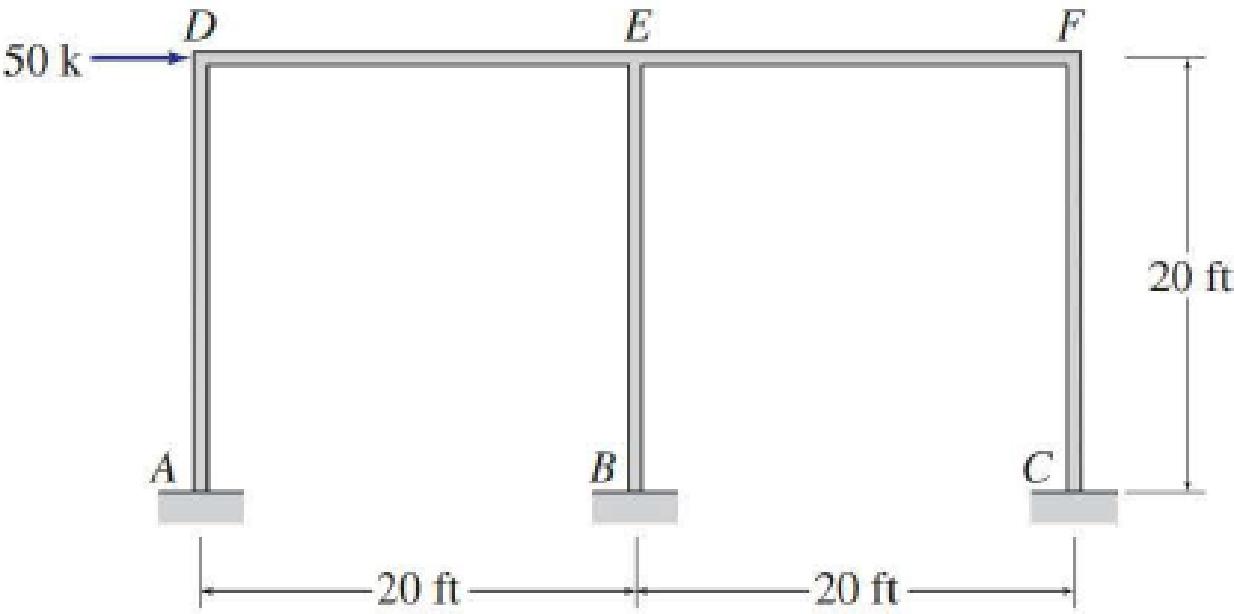# 12.6 through 12.13 Determine the approximate axial forces, shears, and moments for all the members of the frames shown in Figs. P12.6 through P12.13 by using the portal method. FIG. P12.7, P12.15

#### Solutions

Chapter
Section
Chapter 12, Problem 15P
Textbook Problem
7 views

## 12.6 through 12.13 Determine the approximate axial forces, shears, and moments for all the members of the frames shown in Figs. P12.6 through P12.13 by using the portal method.FIG. P12.7, P12.15To determine

Find the approximate axial forces, shears, and moments for the all members of the frames using cantilever method.

### Explanation of Solution

Given information:

The axial force acting at point D (HD) is 50 k.

The vertical distance of the member AD, BE, and CF (L) is 20 ft.

The horizontal distance of the members AB and BC (l1) is 20 ft.

The horizontal distance of the members DE and EF (l2) is 20 ft.

Take the counterclockwise moment is positive and clockwise moment is negative.

The axial force in horizontal direction, towards right is positive and towards left side is negative.

The axial force in vertical direction, towards upward is positive and towards downward is negative.

Calculation:

Insert the internal hinges at the midpoints of all the members of the given frame to obtain the simplified frame for approximate analysis.

Draw the simplified frame as in Figure (1).

For the calculation of column axial forces of story of the frame, pass an imaginary section aa through the internal hinges at the midheights of columns AD, BE, and CF.

Draw the free body diagram of the frame portion with the passed imaginary lines as in Figure (2).

Column axial forces:

Above section aa:

Draw the free body diagram of the frame portion above the section aa as in Figure (3).

Determine the location of the centroid using the relation.

x¯=AxA=Ax1+Ax2+Ax33A (1)

Substitute 0 ft for x1, 20 ft for x2, and 40 ft for x3 in Equation (1).

x¯=A(0)+A(20)+A(40)3A=20ft

The given lateral load is acting on the frame to the right, therefore the axial force in column AD located to the left of the centroid, must be tensile, whereas the axial force in column CF placed to the right of the centroid, must be compressive.

Determine the axial force in the column members AD and CF using equilibrium conditions.

MG=0Q×(l1+l2)HD×L2QBE×l1=0

Substitute 20 ft for l1, 20 ft for l2, 50 k for HD, 20 ft for L, and 0 k for QBE.

Q×(20+20)50×2020=040Q=500Q=50040Q=12.5k

The axial force at the column member AD and CF is QAD=12.5k() and QCF=12.5k().

Draw the free body diagram of the frame portion above the section aa with the axial forces in the column members as in Figure (4).

Girder shear and moments:

Consider girder DE.

Determine the shear at upper left end joint D using equilibrium equation.

SD12.5=0SD=12.5k()

The shear at the girder DE is SDE=12.5k().

Determine the shear at upper right end joint E using equilibrium equation.

FY=0SDE+SED=0

Substitute 12.5 k for SDE.

12.5+SED=0SED=12.5k()

Determine the moment at left end of the girder DE using equilibrium equations.

MDE=SDE×l12

Substitute 12.5 k for SDE and 20 ft for l1.

MDE=12.5×202=125k-ft=125k-ft(Clockwise)

Determine the moment at right end of the girder DE using equilibrium equations.

MD=0MDE+SED×l1MED=0

Substitute 125k-ft for MDE, 12.5 k for SED, and 20 ft for l1.

125+12.5×20MED=0MED=125k-ftMED=125k-ft(Clockwise)

Consider girder EF.

Determine the shear at left end joint E using equilibrium equation.

FY=0SEF+SED=0

Substitute 12.5 k for SED.

SEF+12.5=0SEF=12.5kSEF=12.5k()

Determine the shear at right end joint F using equilibrium equation.

FY=0SEF+SFE=0

Substitute 12.5 k for SEF.

12.5+SFE=0SFE=12.5k()

Determine the moment at left end of the girder EF using equilibrium equations.

MEF=SEF×l22

Substitute 12.5 k for SEF and 20 ft for l2.

MEF=12.5×202=125k-ft=125k-ft(Clockwise)

Determine the moment at right end of the girder EF using equilibrium equations.

ME=0MEF+SFE×l2MFE=0

Substitute 125k-ft for MEF, 12.5 k for SFE, and 20 ft for l2.

125+12.5×20MFE=0MFE=125k-ftMFE=125k-ft(Clockwise)

Column moments and shears:

Column moment for member AD, BE, and CF:

Determine the moment at the column member CE using moment equilibrium of joints.

Apply the moment equilibrium of joints at D.

M=0MDAMDE=0

Substitute 125k-ft for MDE

### Still sussing out bartleby?

Check out a sample textbook solution.

See a sample solution

#### The Solution to Your Study Problems

Bartleby provides explanations to thousands of textbook problems written by our experts, many with advanced degrees!

Get Started

Find more solutions based on key concepts
Which layer of the Earth contains the most amount of mass?

Engineering Fundamentals: An Introduction to Engineering (MindTap Course List)

What are two types of virtual circuits?

Network+ Guide to Networks (MindTap Course List)

What is containment, and why is it part of the planning process?

Principles of Information Security (MindTap Course List)

Convert 5 megawatts of power into BTU/hr, ft-lbs/s, and kJ/hr.

Fundamentals of Chemical Engineering Thermodynamics (MindTap Course List)

What is a telecommunications protocol?

Fundamentals of Information Systems

Define the term, data dictionary. Explain how a data dictionary helps ensure data integrity.

Enhanced Discovering Computers 2017 (Shelly Cashman Series) (MindTap Course List)

If your motherboard supports ECC DDR3 memory, can you substitute non-ECC DDR3 memory?

A+ Guide to Hardware (Standalone Book) (MindTap Course List)

Does all of the heat produced by an SMA weld stay in the weld? Why or why not?

Welding: Principles and Applications (MindTap Course List)